• 随机森林回归
2022-06-02 20:24:32

# 随机森林回归进行调参

提示：以下是本篇文章正文内容，下面案例可供参考

# 一、调参方法

调参过程首先进行依次寻找n_estimators、max_depth、min_samples_split、min_samples_leaf和max_features的最佳参数，然后在最优参数附近进行小范围网格搜索，最终得到最终参数。

# 二、调参过程

导入库并读取数据（ps:加载了很多没用的库-v-）

	from turtle import shape
import pandas as pd
import  pprint
import numpy as np
import scipy.stats as stats
from  sklearn import random_projection
from sklearn.ensemble import RandomForestRegressor
from sklearn.model_selection import RandomizedSearchCV
from sklearn.model_selection import GridSearchCV
import matplotlib.pyplot as plt
from sklearn import metrics
from sklearn.model_selection import train_test_split
from sklearn.model_selection import train_test_split,GridSearchCV,cross_val_score
from sklearn.metrics import accuracy_score
#导入数据

Y_train = Y_train.values.ravel()
# X_train = X_train.values.ravel()

print(X_test.shape)
print(Y_test.shape)
print(Y_train.shape)
print(X_train.shape)


## 1、 调n_estimators参数 :


###调n_estimators参数
ScoreAll = []
for i in range(10,200,1):   #criterion = 'entropy'
DT = RandomForestRegressor(n_estimators = i,random_state = 66)
score = cross_val_score(DT,X_train,Y_train,cv=10).mean()
ScoreAll.append([i,score])
ScoreAll = np.array(ScoreAll)

max_score = np.where(ScoreAll==np.max(ScoreAll[:,1])) ##这句话看似很长的，其实就是找出最高得分对应的索引
print("最优参数以及最高得分:",ScoreAll[max_score])
plt.figure(figsize=[20,5])
plt.plot(ScoreAll[:,0],ScoreAll[:,1])
plt.show()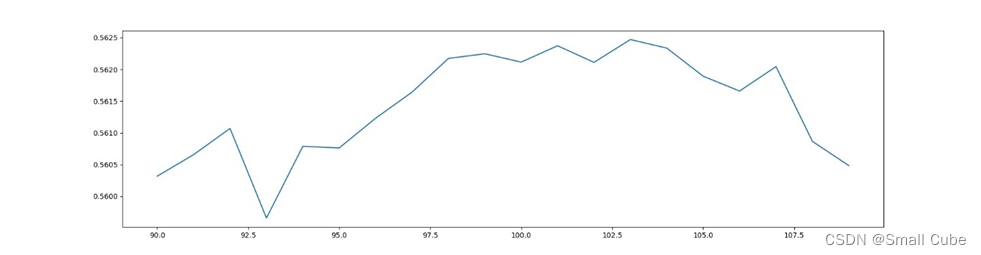可以看到，103为得分最高点，暂定n_estimators为103，接着调下边的参数。

## 2、 探索max_depth的最佳参数

	ScoreAll = []
for i in range(10,30,3):
DT = RandomForestRegressor(n_estimators = 103,random_state = 66,max_depth =i ) #,criterion = 'entropy'
score = cross_val_score(DT,X_train,Y_train,cv=10).mean()
ScoreAll.append([i,score])
ScoreAll = np.array(ScoreAll)

max_score = np.where(ScoreAll==np.max(ScoreAll[:,1]))
print("最优参数以及最高得分:",ScoreAll[max_score])
plt.figure(figsize=[20,5])
plt.plot(ScoreAll[:,0],ScoreAll[:,1])
plt.show()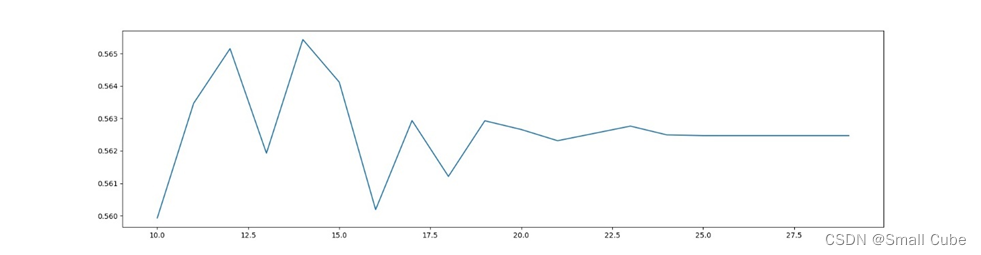根据曲线，暂定树深为14。

## 3、min_samples_split的最佳参数

	ScoreAll = []
for i in range(2,10,1):
DT = RandomForestRegressor(n_estimators = 103,random_state = 66,max_depth =14,min_samples_split = i ) #,criterion = 'entropy'
score = cross_val_score(DT,X_train,Y_train,cv=10).mean()
ScoreAll.append([i,score])
ScoreAll = np.array(ScoreAll)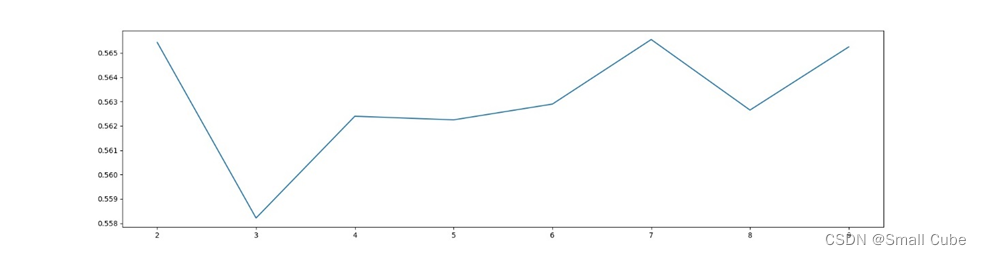从2后模型得分快速下降，后模型得分升高，说明出现了过拟合的情况。故最小样本数选择2

## 4、min_samples_leaf最佳参数

调参代码：

	ScoreAll = []
for i in range(2,10,1):
DT = RandomForestRegressor(n_estimators = 103,random_state = 66,max_depth =14,min_samples_leaf = i,min_samples_split = 2,) #,criterion = 'entropy'
score = cross_val_score(DT,X_train,Y_train,cv=10).mean()
ScoreAll.append([i,score])
ScoreAll = np.array(ScoreAll)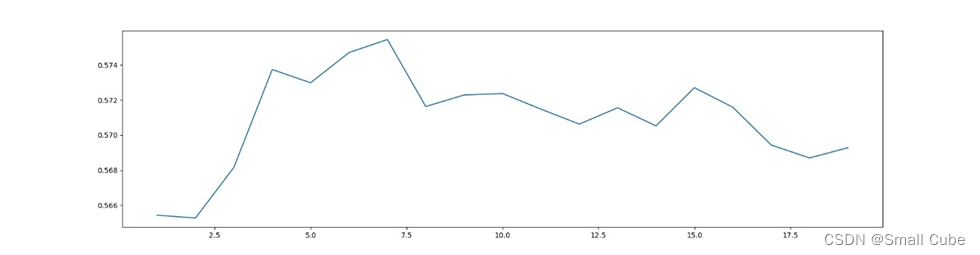根据曲线显示，最小叶子数选为7

## 5、max_features调参

	param_grid = {
'max_features':np.arange(0.1, 1)}
rfc = RandomForestRegressor(random_state=66,n_estimators = 103,max_depth = 14,min_samples_leaf =7 ,min_samples_split =2 )
GS = GridSearchCV(rfc,param_grid,cv=10)
GS.fit(X_train,Y_train)
print(GS.best_params_)
print(GS.best_score_)



## 6、小范围修改

由于 min_samples_leaf 和min_samples_split会相互影像，故把这三个参数一起网格搜索：

	param_grid = {
'max_features':np.arange(0.1, 1),
'min_samples_leaf':np.arange(5,15),
'min_samples_split':np.arange(2,10),
}
rfc = RandomForestRegressor(random_state=66,n_estimators = 103,max_depth = 14 )
GS = GridSearchCV(rfc,param_grid,cv=10)
GS.fit(X_train,Y_train)
print(GS.best_params_)
print(GS.best_score_)



结果：

{'max_features': 0.1,
'min_samples_leaf': 5,
'min_samples_split': 2}
0.5697799544764119


参数值:

n_estimators103
max_depth14
max_features0.1
min_samples_leaf5
min_samples_split2

## 7、在得到的最优参数附近进行小范围网格搜索

	import time
start = time.time()
param_grid = {
'n_estimators':np.arange(100, 110),
'max_depth':np.arange(12, 16),
'min_samples_leaf':np.arange(1, 8),
'min_samples_split':np.arange(2, 5),
'max_features':np.arange(0.1, 1)
}

rfc = RandomForestRegressor(random_state=66)
GS = GridSearchCV(rfc,param_grid,cv=10)
GS.fit(X_train,Y_train)
end = time.time()
print("循环运行时间:%.2f秒"%(end-start))
print(GS.best_params_)
print(GS.best_score_)



结果：

参数旧值新值
n_estimators103106
max_depth1414
max_features 0.10.1
min_samples_leaf51
min_samples_split23

## 8、随机森林回归预测

def test_RandomForestRegressor(X_train,X_test,Y_train,Y_test):
regr=RandomForestRegressor(n_estimators=103,max_depth=14,min_samples_split=3,min_samples_leaf=1,max_features=0.1)
regr.fit(X_train,Y_train)
predictions = regr.predict(X_test)
data = pd.DataFrame(predictions)
print("树木个数:103,max_depth=14,split=2,min_samples_split=3,min_samples_leaf=1,max_feature=0.1","Traing Score:%f"%regr.score(X_train,Y_train),"Testing Score:%f"%regr.score(X_test,Y_test))
predictions=regr.predict(X_test)
# plt.plot(Y_test['date'], predictions['predictionspredictions'], 'ro', label = 'prediction')
# print(predictions)
writer = pd.ExcelWriter("D:\科研\RandomForest\新建文件夹\predict06.xlsx")      # 写入Excel文件
data.to_excel(writer,sheet_name='num',na_rep='nana',index=False)        # ‘page_1’是写入excel的sheet名
writer.save()
writer.close()

test_RandomForestRegressor(X_train,X_test,Y_train,Y_test)


感谢：本文调参参考了疯狂学GIS的博文，从中学习颇多。https://blog.csdn.net/zhebushibiaoshifu/category_10827929.html?spm=1001.2014.3001.5515sklearn
更多相关内容
• 随机森林是一种基于分类树的算法，这个算法需要模拟和迭代，被归类为机器学习中的一种方法。
• matlab软件随机森林回归模型代码，可直接matlab打开运行！精简版，包括数据导入、模型建立、误差计算、保存模型，绘制对比图的多个功能！
• 主要利用R语言进行随机森林回归，还有其他两种回归， library(lattice) library(grid) library(DMwR) library(rpart) library(ipred) library(randomForest) #回归树，装袋算法，随机森林三大回归 #前二种算法可以...
• 随机森林回归matlab代码，可用于回归和分类，简单易用
• 随机森林算法随机森林是一种比较新的机器学习模型。
• RF_regressor 使用sklearn随机森林回归器的预测模型
• 随机森林回归 建模 数据分析 matlab RF
• 随机森林回归中寻找最佳mtry和ntree.txt
• 通过随机森林回归分析自动提取3D形状的通用焦点特征研究论文
• 资料说明：包括数据集+源代码+Word文档说明。 资料内容包括： 1)问题定义； 2)获取数据； 3)数据预处理； 4)探索性数据分析； 5)特征工程； 6)机器建模； 7)模型评估； 8)实际应用。
• 主要通过sklearn中来实现随机森林回归模型对波士顿房价进行回归预测
• #Get data for tarfile in Github, extract it in datasets/housing:import osimport tarfilefrom six . moves import urllibDOWNLOAD_ROOT = ... And save in datasets/housingdef getdata
• 我使用“加利福尼亚房屋价格数据集”的“随机森林回归”建立了一个模型，以预测加利福尼亚房屋的价格。 图书馆与依存关系 我在这里列出了该项目所需的所有必要的库和依赖项： import sys , os , tarfile , urllib ...
• 通过建立随机森林，实现大数据样本拟合回归随机森林参数通过误差最低确定
• 随机森林回归，以及回归的精度分析和评价，MATLAB
•  2 随机森林回归器的参数   2.1 弱分类器结构   2.2 弱分类器数量   2.3 弱分类器训练的数据   2.4 其他参数 四 增量学习：随机森林处理巨量数据  1 普通学习vs增量学习  2 增量学习在Kaggle数据上的应用...

目录

三 随机森林RandomForest
1 RamdomForestRegressor的实现
2 随机森林回归器的参数
2.1 弱分类器结构
2.2 弱分类器数量
2.3 弱分类器训练的数据
2.4 其他参数
四 增量学习：随机森林处理巨量数据
1 普通学习vs增量学习
2 增量学习在Kaggle数据上的应用
五 原理进阶：Bagging方法6大面试热点问题
六 随机森林的参数空间与自动优化

# 三 随机森林RandomForest

随机森林是机器学习领域最常用的算法之一，其算法构筑过程非常简单：从提供的数据中随机抽样出不同的子集，用于建立多棵不同的决策树，并按照Bagging的规则对单棵决策树的结果进行集成（回归则平均，分类则少数服从多数）。只要你充分掌握了决策树的各项属性和参数，随机森林的大部分内容都相当容易理解。

虽然原理上很简单，但随机森林的学习能力异常强大、算法复杂度高、又具备一定的抗过拟合能力，是从根本上来说比单棵决策树更优越的算法。即便在深入了解机器学习的各种技巧之后，它依然是我们能够使用的最强大的算法之一。原理如此简单、还如此强大的算法在机器学习的世界中是不常见的。在机器学习竞赛当中，随机森林往往是我们在中小型数据上会尝试的第一个算法

在sklearn中，随机森林可以实现回归也可以实现分类。随机森林回归器由类sklearn.ensemble.RandomForestRegressor实现，随机森林分类器则有类sklearn.ensemble.RandomForestClassifier实现。我们可以像调用逻辑回归、决策树等其他sklearn中的算法一样，使用“实例化、fit、predict/score”三部曲来使用随机森林，同时我们也可以使用sklearn中的交叉验证方法来实现随机森林。其中回归森林的默认评估指标为R2，分类森林的默认评估指标为准确率。

class sklearn.ensemble.RandomForestRegressor(n_estimators=100, *, criterion='squared_error', max_depth=None, min_samples_split=2, min_samples_leaf=1, min_weight_fraction_leaf=0.0, max_features='auto', max_leaf_nodes=None, min_impurity_decrease=0.0, bootstrap=True, oob_score=False, n_jobs=None, random_state=None, verbose=0, warm_start=False, ccp_alpha=0.0, max_samples=None)

class sklearn.ensemble.RandomForestClassifier(n_estimators=100, *, criterion='gini', max_depth=None, min_samples_split=2, min_samples_leaf=1, min_weight_fraction_leaf=0.0, max_features='auto', max_leaf_nodes=None, min_impurity_decrease=0.0, bootstrap=True, oob_score=False, n_jobs=None, random_state=None, verbose=0, warm_start=False, class_weight=None, ccp_alpha=0.0, max_samples=None)

不难发现，随机森林回归器和分类器的参数高度一致，因此我们只需要讲解其中一个类即可。任意集成算法在发源时都是回归类算法，因此我们的重点将会放在回归类算法上。随机森林有大量的参数，幸运的是，随机森林中所有参数都有默认值，因此即便我们不学习任何参数，也可以调用随机森林算法。我们先来建一片森林看看吧：

## 1 RandomForestRegressor的实现

import matplotlib.pyplot as plt
from sklearn.ensemble import RandomForestRegressor as RFR
from sklearn.tree import DecisionTreeRegressor as DTR
from sklearn.model_selection import cross_validate, KFold
#这里我们不再使用cross_val_score，转而使用能够输出训练集分数的cross_validate
#决策树本身就是非常容易过拟合的算法，而集成模型的参数量/复杂度很难支持大规模网格搜索
#因此对于随机森林来说，一定要关注算法的过拟合情况

data.head()

结果：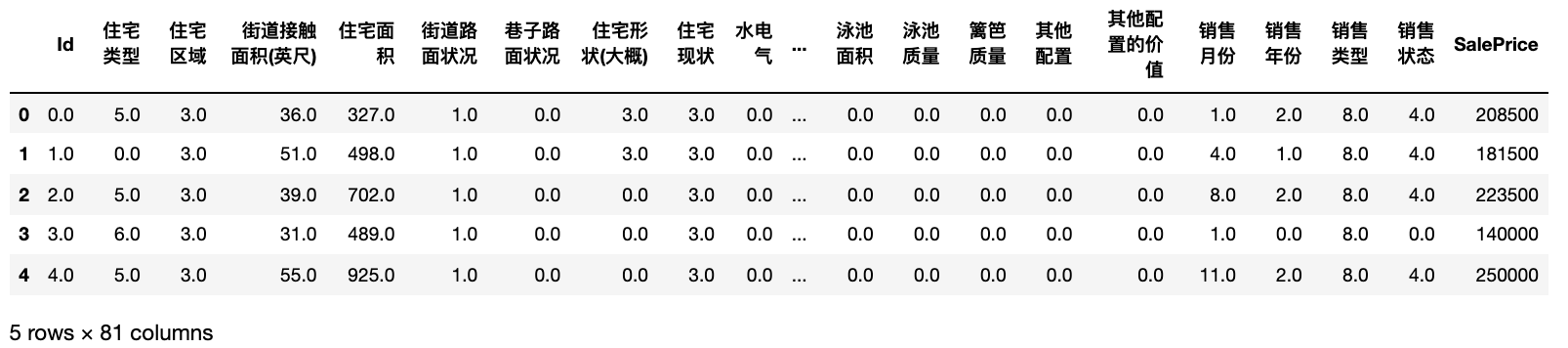data.shape
#(1460, 81)

X = data.iloc[:,:-1]
y = data.iloc[:,-1]

y #注意，y的类型是整数型，并且y的均值很大，可想而知整体的MSE一定会很大
#0       208500
#1       181500
#2       223500
#3       140000
#4       250000
#         ...
#1455    175000
#1456    210000
#1457    266500
#1458    142125
#1459    147500
#Name: SalePrice, Length: 1460, dtype: int64

y.mean()
#180921.19589041095

X.shape
#(1460, 80)

X.columns.tolist()
#['Id',
# '住宅类型',
# '住宅区域',
# '街道接触面积(英尺)',
# '住宅面积',
# '街道路面状况',
# '巷子路面状况',...]

与sklearn中其他回归算法一样，随机森林的默认评估指标是R2，但在机器学习竞赛、甚至实际使用时，我们很少使用损失以外的指标对回归类算法进行评估。对回归类算法而言，最常见的损失就是MSE。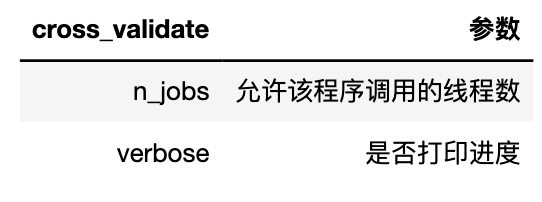reg_f = RFR() #实例化随机森林
reg_t = DTR() #实例化决策树
cv = KFold(n_splits=5,shuffle=True,random_state=1412) #实例化交叉验证方式

result_t = cross_validate(reg_t #要进行交叉验证的评估器
,X,y #数据
,cv=cv #交叉验证模式
,scoring="neg_mean_squared_error"
#评估指标,sklearn中默认为负，负的程度越深模型越糟糕
,return_train_score=True #是否返回训练分数
,verbose=True #是否打印进程
,n_jobs=-1 #线程数
)
#[Parallel(n_jobs=-1)]: Using backend LokyBackend with 8 concurrent workers.
#[Parallel(n_jobs=-1)]: Done   2 out of   5 | elapsed:    1.1s remaining:    1.6s
#[Parallel(n_jobs=-1)]: Done   5 out of   5 | elapsed:    1.1s finished
result_f = cross_validate(reg_f,X,y,cv=cv,scoring="neg_mean_squared_error"
,return_train_score=True
,verbose=True
,n_jobs=-1)
#[Parallel(n_jobs=-1)]: Using backend LokyBackend with 8 concurrent workers.
#[Parallel(n_jobs=-1)]: Done   2 out of   5 | elapsed:    1.4s remaining:    2.1s
#[Parallel(n_jobs=-1)]: Done   5 out of   5 | elapsed:    2.1s finished
'''建立了100颗树，但由于树是并行增长的，所以建立只会比决策树慢一点，随着数据量增长，
随机森林会越来越慢'''

result_t #超级过拟合
# {'fit_time': array([0.03513908, 0.02617216, 0.02826715, 0.03085804, 0.03072166]),
#  'score_time': array([0.00184107, 0.00152969, 0.00140595, 0.00212121, 0.00243092]),
#  'test_score': array([-1.32676659e+09, -3.30251909e+09, -1.57903793e+09, -1.50256213e+09,
#         -1.57774956e+09]),
#  'train_score': array([-0., -0., -0., -0., -0.])}

result_f #训练集和测试在交叉验证上的分数差异更小，因此森林的过拟合程度没有决策树高
# {'fit_time': array([1.30783272, 1.37094092, 1.34987903, 1.40098619, 1.32293487]),
#  'score_time': array([0.00880313, 0.00958204, 0.00925899, 0.00892091, 0.00915027]),
#  'test_score': array([-7.78513095e+08, -2.03928947e+09, -7.46760247e+08, -4.73092198e+08,
#         -8.86053174e+08]),
#  'train_score': array([-1.22052661e+08, -1.04873049e+08, -1.37851525e+08, -1.30651561e+08,
#         -1.26205314e+08])}

在集成学习中，我们衡量回归类算法的指标一般是RMSE（根均方误差），也就是MSE开根号后的结果。现实数据的标签往往数字巨大、数据量庞杂，MSE作为平方结果会放大现实数据上的误差（例如随机森林结果中得到的，7∗1087∗108等结果），因此我们会对平房结果开根号，让回归类算法的评估指标在数值上不要过于夸张。同样的，方差作为平方结果，在现实数据上也会太大，因此如果可以，我们使用标准差进行模型稳定性的衡量。

trainRMSE_f = abs(result_f["train_score"])**0.5
testRMSE_f = abs(result_f["test_score"])**0.5
trainRMSE_t = abs(result_t["train_score"])**0.5
testRMSE_t = abs(result_t["test_score"])**0.5

trainRMSE_f.mean()
#10949.819569879735
testRMSE_f.mean()
#30452.218280217272
trainRMSE_f.std() #方差数额太大，使用标准差
#373.26874603409686

#默认值下随机森林的RMSE与标准差std
xaxis = range(1,6)
plt.figure(figsize=(8,6),dpi=80)
#RMSE
plt.plot(xaxis,trainRMSE_f,color="green",label = "RandomForestTrain")
plt.plot(xaxis,testRMSE_f,color="green",linestyle="--",label = "RandomForestTest")
plt.plot(xaxis,trainRMSE_t,color="orange",label = "DecisionTreeTrain")
plt.plot(xaxis,testRMSE_t,color="orange",linestyle="--",label = "DecisionTreeTest")
plt.xticks([1,2,3,4,5])
plt.xlabel("CVcounts",fontsize=16)
plt.ylabel("RMSE",fontsize=16)
plt.legend()
plt.show()

结果：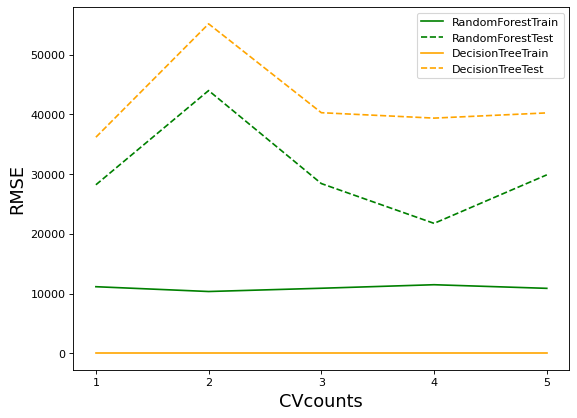• 横坐标：交叉验证次数
• 纵坐标：RMSE数值

从图像来看，森林与决策树都处于过拟合状态，不过森林的过拟合程度较轻，决策树的过拟合程度较强。两个算法在训练集上的结果都比较优秀，决策树的可以完美学习训练集上的内容，达到RMSE=0的程度，而随机森林在训练集上的RMSE大约在1w上下徘徊，测试集上的结果则是随机森林更占优。可见，与填写的参数无关，随机森林天生就是比决策树更不容易过拟合、泛化能力更强的。

## 2 随机森林回归器的参数

当填写参数之后，随机森林可以变得更强大。比起经典机器学习算法逻辑回归、岭回归等，随机森林回归器的参数数量较多，因此我们可以将随机森林类的参数分为如下4大类别，其中标注为绿色的是我们从未学过的、只与集成算法相关的参数：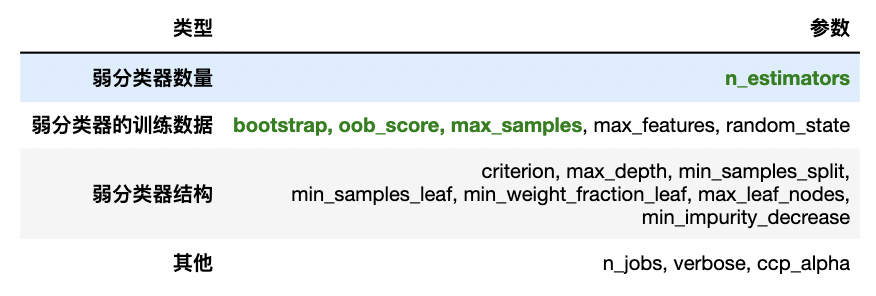### 2.1 弱分类器结构

在集成算法当中，控制单个弱评估器的结构是一个重要的课题，因为单个弱评估器的复杂度/结果都会影响全局，其中单棵决策树的结构越复杂，集成算法的整体复杂度会更高，计算会更加缓慢、模型也会更加容易过拟合，因此集成算法中的弱评估器也需要被剪枝。随机森林回归器的弱评估器是回归树，因此集成评估器中有大量的参数都与弱评估器回归树中的参数重合：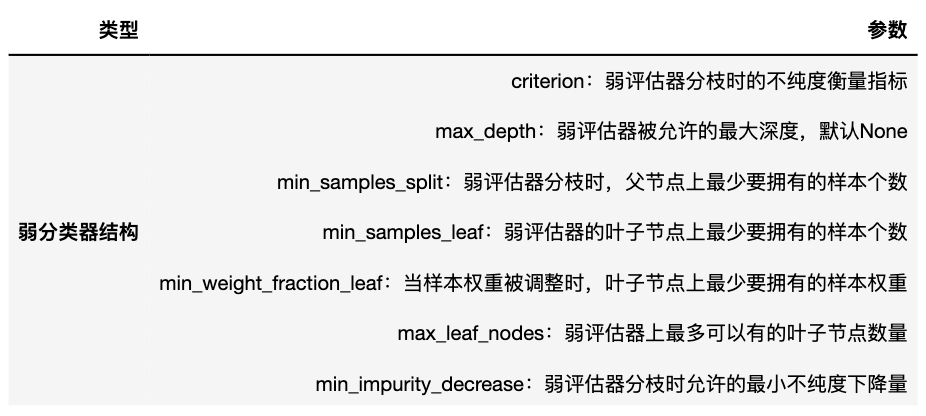这些参数在随机森林中的用法与默认值与决策树类DecisionTreeRegressor中完全一致，专门用于对决策树进行剪枝、控制单个弱评估器的结构，考虑到大家在决策树中已经充分掌握这些参数，我们不再对这些参数一一进行详细说明了。在这里，我们重点复习一下以下两部分参数：

• 分枝标准与特征重要性

criterion与feature_importances_

与分类树中的信息熵/基尼系数不同，回归树中的criterion可以选择"squared_error"（平方误差），"absolute_error"（绝对误差）以及"poisson"（泊松偏差）。对任意样本𝑖而言，𝑦𝑖为真实标签，𝑦𝑖帽为预测标签，则各个criterion的表达式为：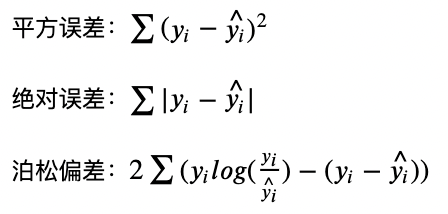其中平方误差与绝对误差是大家非常熟悉的概念，作为分枝标准，平方误差比绝对误差更敏感（类似于信息熵比基尼系数更敏感），并且在计算上平方误差比绝对误差快很多。泊松偏差则是适用于一个特殊场景的：当需要预测的标签全部为正整数时，标签的分布可以被认为是类似于泊松分布的。正整数预测在实际应用中非常常见，比如预测点击量、预测客户/离职人数、预测销售量等。我们现在正在使用的数据（房价预测），也可能比较适合于泊松偏差。

另外，当我们选择不同的criterion之后，决策树的feature_importances_也会随之变化，因为在sklearn当中，feature_importances_是特征对criterion下降量的总贡献量，因此不同的criterion可能得到不同的特征重要性。（criterion和feature_importances_相连，feature_importances_是通过每一个特征贡献的不纯度下降量计算的

对我们来说，选择criterion的唯一指标就是最终的交叉验证结果——无论理论是如何说明的，我们只取令随机森林的预测结果最好的criterion。

• 调节树结构来控制过拟合

max_depth

最粗犷的剪枝方式，从树结构层面来看，对随机森林抗过拟合能力影响最大的参数。max_depth的默认值为None，也就是不限深度。因此当随机森林表现为过拟合时，选择一个小的max_depth会很有效。

max_leaf_nodes与min_sample_split

比max_depth更精细的减枝方式，但限制叶子数量和分枝，既可以实现微调，也可以实现大刀阔斧的剪枝。max_leaf_nodes的默认值为None，即不限叶子数量。min_sample_split的默认值为2，等同于不限制分枝。

min_impurity_decrease

最精细的减枝方式，可以根据不纯度下降的程度减掉相应的叶子。默认值为0，因此是个相当有空间的参数。

### 2.2 弱分类器数量

n_estimators

n_estimators是森林中树木的数量，即弱评估器的数量，在sklearn中默认100，它是唯一一个对随机森林而言必填的参数。n_estimators对随机森林模型的精确程度、复杂度、学习能力、过拟合情况、需要的计算量和计算时间都有很大的影响，因此n_estimators往往是我们在调整随机森林时第一个需要确认的参数。对单一决策树而言，模型复杂度由树结构（树深、树宽、树上的叶子数量等）与数据量（样本量、特征量）决定，而对随机森林而言，模型复杂度由森林中树的数量、树结构与数据量决定，其中树的数量越多，模型越复杂。

还记得讲解决策树与逻辑回归时我们绘制的这张图像么？当模型复杂度上升时，模型的泛化能力会先增加再下降（相对的泛化误差会先下降再上升），我们需要找到模型泛化能力最佳的复杂度。在实际进行训练时，最佳复杂度往往是一个比较明显的转折点，当复杂度高于最佳复杂度时，模型的泛化误差要么开始上升，要么不再下降。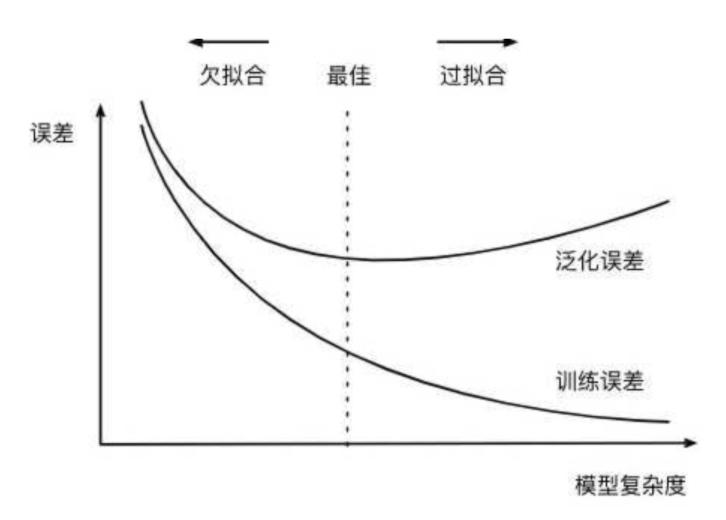对随机森林而言，该图像的横坐标可以被无缝切换为参数n_estimators上的值。当n_estimators越大时：

• 模型的复杂程度上升，泛化能先增强再减弱（或不变）
• 模型的学习能力越来越强，在训练集上的分数可能越来越高，过拟合风险越来越高
• 模型需要的算力和内存越来越多
• 模型训练的时间会越来越长

因此在调整n_estimators时，我们总是渴望在模型效果与训练难度之间取得平衡，同时我们还需要使用交叉验证来随时关注模型过拟合的情况。在sklearn现在的版本中，n_estimators的默认值为100，个人电脑能够容忍的n_estimators数量大约在200~1000左右。

def RMSE(cvresult,key):
return (abs(cvresult[key])**0.5).mean()

reg_f = RFR(n_estimators=3)
cv = KFold(n_splits=5,shuffle=True,random_state=1412)
result_f = cross_validate(reg_f,X,y,cv=cv,scoring="neg_mean_squared_error"
,return_train_score=True
,verbose=True
,n_jobs=-1)
#[Parallel(n_jobs=-1)]: Using backend LokyBackend with 8 concurrent workers.
#[Parallel(n_jobs=-1)]: Done   2 out of   5 | elapsed:    1.3s remaining:    1.9s
#[Parallel(n_jobs=-1)]: Done   5 out of   5 | elapsed:    1.3s finished
RMSE(result_f,"test_score")
#35681.96994493137

reg_f = RFR(n_estimators=100)
cv = KFold(n_splits=5,shuffle=True,random_state=1412)
result_f = cross_validate(reg_f,X,y,cv=cv,scoring="neg_mean_squared_error"
,return_train_score=True
,verbose=True
,n_jobs=-1)
#[Parallel(n_jobs=-1)]: Using backend LokyBackend with 8 concurrent workers.
#[Parallel(n_jobs=-1)]: Done   2 out of   5 | elapsed:    1.4s remaining:    2.1s
#[Parallel(n_jobs=-1)]: Done   5 out of   5 | elapsed:    2.0s finished
RMSE(result_f,"test_score")
#30351.359534374766

reg_f = RFR(n_estimators=500)
cv = KFold(n_splits=5,shuffle=True,random_state=1412)
result_f = cross_validate(reg_f,X,y,cv=cv,scoring="neg_mean_squared_error"
,return_train_score=True
,verbose=True
,n_jobs=-1)
#[Parallel(n_jobs=-1)]: Using backend LokyBackend with 8 concurrent workers.
#[Parallel(n_jobs=-1)]: Done   2 out of   5 | elapsed:    7.8s remaining:   11.8s
#[Parallel(n_jobs=-1)]: Done   5 out of   5 | elapsed:    7.9s finished
RMSE(result_f,"test_score")
#30290.45615417552



随机森林bagging生成的树都是独立并行计算的，所以时间不会成倍增加

总结：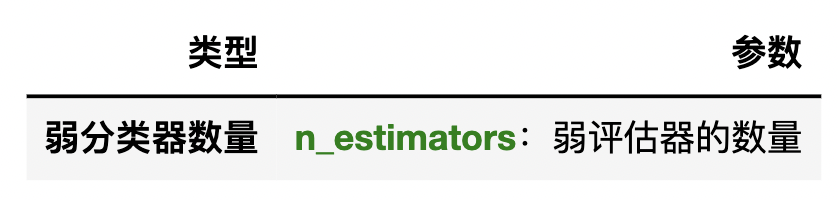### 2.3 弱分类器训练的数据

还记得决策树是如何分枝的吗？对每个特征决策树都会找到不纯度下降程度最大的节点进行分枝，因此原则上来说，只要给出数据一致、并且不对决策树进行减枝的话，决策树的结构一定是完全相同的。对集成算法来说，平均多棵相同的决策树的结果并没有意义，因此集成算法中每棵树必然是不同的树，Bagging算法是依赖于随机抽样数据来实现这一点的。

随机森林会从提供的数据中随机抽样出不同的子集，用于建立多棵不同的决策树，最终再按照Bagging的规则对众多决策树的结果进行集成。因此在随机森林回归器的参数当中，有数个关于数据随机抽样的参数。

• 样本的随机抽样

bootstrap，oob_score，max_samples

bootstrap参数的输入为布尔值，默认True，控制是否在每次建立决策树之前对数据进行随机抽样。如果设置为False，则表示每次都使用全部样本进行建树，如果为True，则随机抽样建树。从语言的意义上来看，bootstrap可以指代任意类型的随机抽样，但在随机森林中它特指有放回随机抽样技术

如下图所示，在一个含有m个样本的原始训练集中，我们进行随机采样。每次采样一个样本，并在抽取下一个样本之前将该样本放回原始训练集，也就是说下次采样时这个样本依然可能被采集到，这样采集max_samples次，最终得到max_samples个样本组成的自助集。

自助集就是抽取出来的数据集。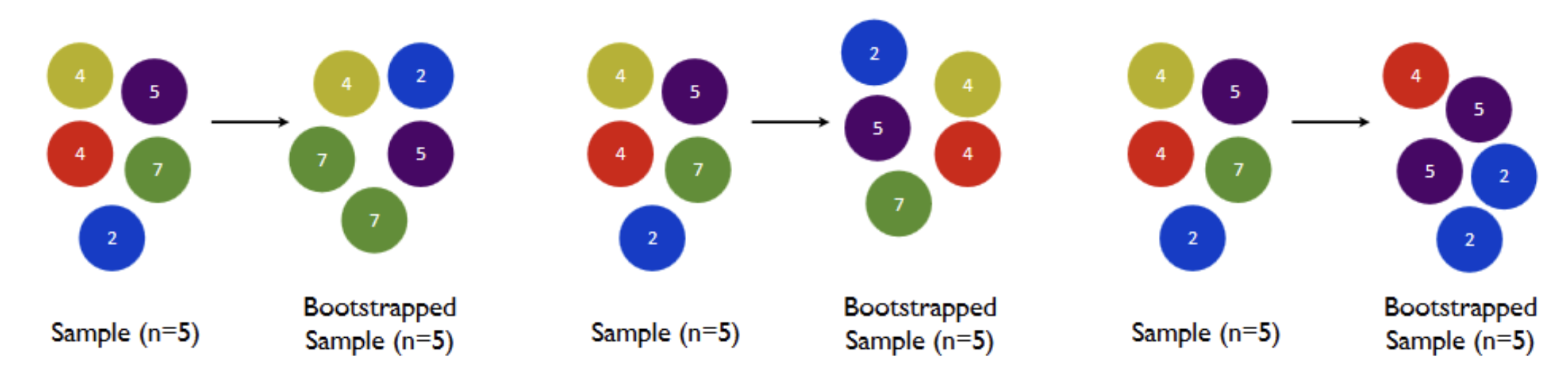通常来说，max_samples是等于m的（行业惯例），也就是抽样数据集的大小与原始数据集一致，但是如果原始数据集太大、或者太小，我们也可以自由调整max_samples的大小。由于是随机采样，这样每次的自助集和原始数据集不同，和其他的采样集也是不同的。这样我们就可以自由创造取之不尽用之不竭，并且互不相同的自助集，用这些自助集来训练我们的弱分类器，我们的弱分类器自然也就各不相同了。

然而有放回抽样也会有自己的问题。由于是有放回，一些样本可能在同一个自助集中出现多次，而其他一些却可能被忽略。当抽样次数足够多、且原始数据集足够大时，自助集大约平均会包含全数据的63%，这个数字是有数学依据的。因为在max_samples次抽样中，一个样本被抽到某个自助集中的概率为：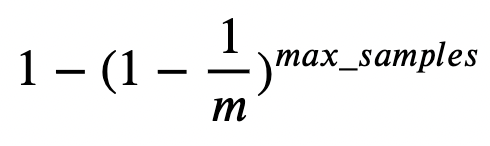这个式子是怎么来的呢？对于任意一个样本而言：

一次抽样时抽到该样本的概率为1/m

一次抽样时抽不到该样本的概率为1−1/m

总共抽样max_samples次，一次也没有抽到该样本的概率就是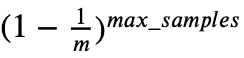因此1减去该概率，就是一个样本在抽样中一定会被抽到某个自助集的概率。 当m刚好等于max_samples时，公式可以被修改为：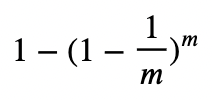这明显是一个经典的极限问题，由洛必达法则（L'Hôpital's rule）我们可知：当m足够大时（接近极限时），这个概率收敛于1-(1/e)，其中e是自然常数，整体概率约等于0.632。因此，会有约37%的训练数据被浪费掉，没有参与建模，这些数据被称为袋外数据(out of bag data，简写为oob)。在实际使用随机森林时，袋外数据常常被我们当做验证集使用，所以我们或许可以不做交叉验证、不分割数据集，而只依赖于袋外数据来测试我们的模型即可。当然，这也不是绝对的，当树的数量n_estimators不足，或者max_samples太小时，很可能就没有数据掉落在袋外，自然也有无法使用oob数据来作为验证集了。

在随机森林回归器中，当boostrap=True时，我们可以使用参数oob_scoremax_samples，其中：

oob_score控制是否使用袋外数据进行验证，输入为布尔值，默认为False，如果希望使用袋外数据进行验证，修改为True即可。（数据量特别大的时候，可以修改为True）

max_samples表示自助集的大小，可以输入整数、浮点数或None，默认为None。

输入整数m，则代表每次从全数据集中有放回抽样m个样本
输入浮点数f，则表示每次从全数据集中有放回抽样f*全数据量个样本
输入None，则表示每次抽样都抽取与全数据集一致的样本量（X.shape）

在使用袋外数据时，我们可以用随机森林的另一个重要属性：oob_score_来查看我们的在袋外数据上测试的结果，遗憾的是我们无法调整oob_score_输出的评估指标，它默认是R2。

reg = RFR(n_estimators=20
, bootstrap=True #进行随机抽样
, oob_score=True #按袋外数据进行验证
, max_samples=500
).fit(X,y)
#重要属性oob_score_
reg.oob_score_ #在袋外数据上的R2为83%
#0.8379331878464579

reg = RFR(n_estimators=20
, bootstrap=False
, oob_score=True
, max_samples=500).fit(X,y)
#直接无法运行，因为boostrap=False时oob_score分数根本不存在

reg = RFR(n_estimators=20
, bootstrap=True #允许抽样
, oob_score=False #但不进行计算
, max_samples=500).fit(X,y)
reg.oob_score_ #虽然可以训练，但oob_score_无法被调用
• 特征的随机抽样

max_features

数据抽样还有另一个维度：对特征的抽样。在学习决策树时，我们已经学习过对特征进行抽样的参数max_features，在随机森林中max_features的用法与决策树中完全一致，其输入也与决策树完全一致：

输入整数，表示每次分枝时随机抽取max_features个特征
输入浮点数，表示每次分枝时抽取round(max_features * n_features)个特征
输入"auto"或者None，表示每次分枝时使用全部特征n_features
输入"sqrt"，表示每次分枝时使用sqrt(n_features)
输入"log2"，表示每次分枝时使用log2(n_features)

sqrt_ = []
log_ = []
for n_features in range(1,101,2):
sqrt_.append(np.sqrt(n_features))
log_.append(np.log2(n_features))
xaxis = range(1,101,2)
plt.figure(figsize=(8,6),dpi=80)
#RMSE
plt.plot(xaxis,sqrt_,color="green",label = "sqrt(n)")
plt.plot(xaxis,log_,color="orange",label = "log2(n)")
plt.xticks(range(1,101,10))
plt.legend()
plt.show()

结果：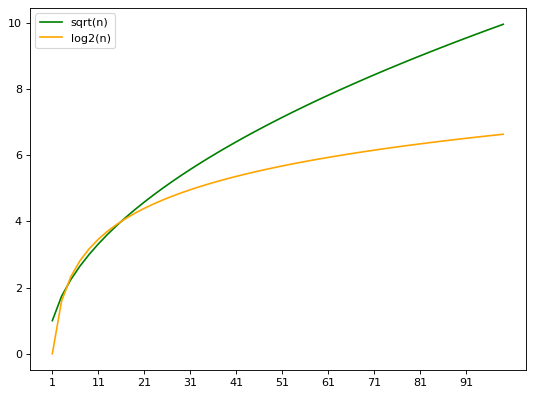不难发现，sqrt(n_features)和log2(n_features)都会返回一个比原始特征量小很多的数，但一般情况下log2返回的值比sqrt返回的值更小，因此如果我们想要树之间的差异更大，我们可以设置模式为log2。在实际使用时，我们往往会先使用上述的文字输入，观察模型的结果，然后再在有效的范围附近进行网格搜索。

需要注意的是，无论对数据进行怎样的抽样，我们能够控制的都只是建立单棵树时的数据而已。在总数据量有限的情况下，单棵树使用的数据量越大，每一棵树使用的数据就会越相似，每棵树的结构也就会越相似，bagging的效果难以发挥、模型也很容易变得过拟合。因此，当数据量足够时，我们往往会消减单棵树使用的数据量。

• 随机抽样的模式

random_state

在决策树当中，我们已经学习过控制随机模式的参数random_state，这个参数是“随机数种子”，它控制决策树当中多个具有随机性的流程。在sklearn实现的随机森林当中，决策树上也存在众多有随机性的流程：

• 「强制」随机抽取每棵树建立时分枝用的特征，抽取的数量可由参数max_features决定
• 「强制」随机排序每棵树分枝时所用的特征（工业上不可能一个一个算）
• 「可选」随机抽取每棵树建立时训练用的样本，抽取的比例可由参数max_samples决定

因此每次使用随机森林类时，我们建立的集成算法都是不同的，在同一个数据集上多次建树自然也会产生不同的模型结果。因此在工程部署和教学当中，我们在建树的第一步总是会先设置随机数种子为一个固定值，让算法固定下来。在设置的时候，需要注意两个问题：

1、不同库中的随机数种子遵循不同的规则，对不同库中的随机数种子给与相同的数字，也不会得到相同的结果

import pandas as pd
import random
list_ = [1,2,3,4,5]
list_p = pd.Series(list_)
list_p
#0    1
#1    2
#2    3
#3    4
#4    5
#dtype: int64

#random中的随机抽样
random.seed(1)
random.sample(list_,k=3)
#[2, 1, 5]

#pandas中的随机抽样
list_p.sample(n=3,random_state=1).values
#array([3, 2, 5])

同样的，sklearn中的随机抽样、numpy中的随机抽样、cuda中的随机抽样在相同的随机数种子数值下，都会得到不同的结果。

2、如何选择最佳随机数种子？

当数据样本量足够大的时候（数万），变换随机数种子几乎不会对模型的泛化能力有影响，因此在数据量巨大的情况下，我们可以随意设置任意的数值。

当数据量较小的时候，我们可以把随机数种子当做参数进行调整，但前提是必须依赖于交叉验证的结果。选择交叉验证结果中均值最高、方差最低的随机数种子，以找到泛化能力最强大的随机模式。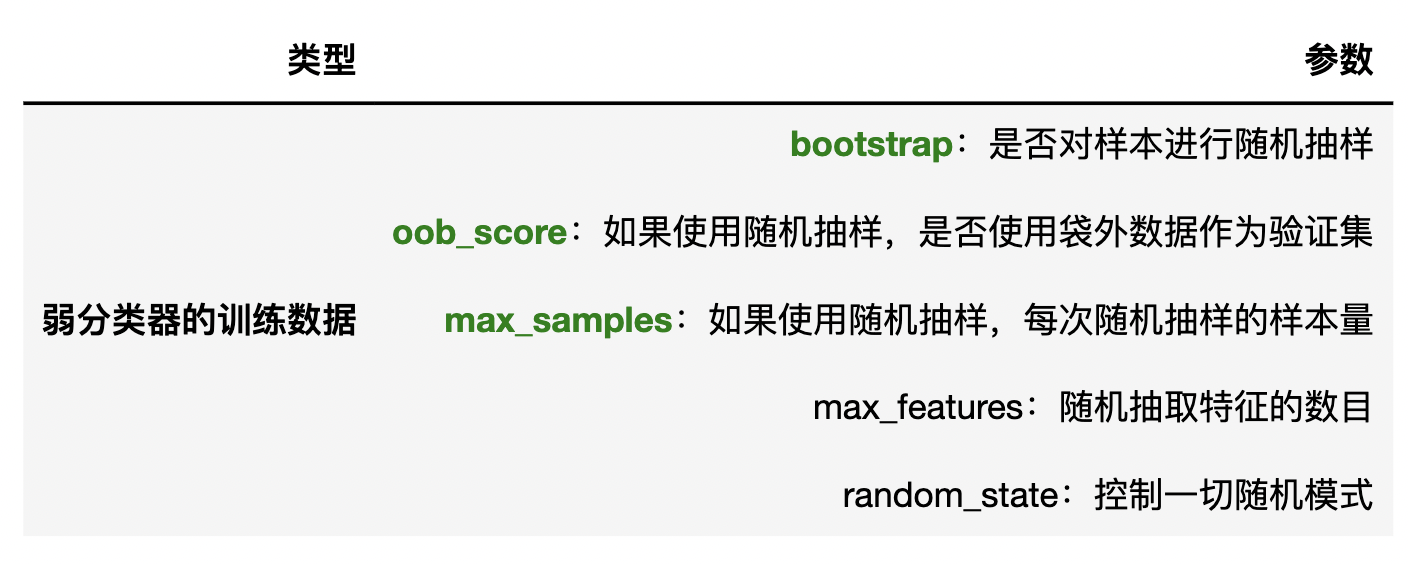### 2.4 其他参数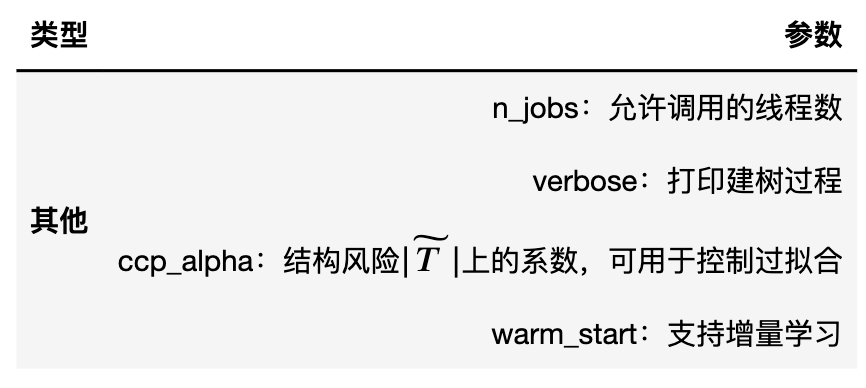我们已经了解过前三个参数。需要稍微说明一下verbose参数。随机森林的verbose参数打印的是建树过程，但只有在树的数量众多、建模耗时很长时，verbose才会打印建树的具体过程，否则它只会打印出一行两简单的报告。这些参数中需要重点说明的是warm_startwarm_start是控制增量学习的参数，默认为False，该参数可以帮助随机森林处理巨量数据，解决围绕随机森林的众多关键问题。我们将在之后的章节中重点讲解warm_start的应用。

展开全文算法 机器学习
• oob_score三、重要属性和接口四、随机森林回归器1、重要参数，属性与接口2、实例：用随机森林回归填补缺失值五、机器学习中调参的基本思想六、 实例：随机森林在乳腺癌数据上的调参 一、集成算法概述 集成学习...

# 一、集成算法概述

集成学习（ensemble learning）是时下非常流行的机器学习算法，它本身不是一个单独的机器学习算法，而是通过在数据上构建多个模型，集成所有模型的建模结果。基本上所有的机器学习领域都可以看到集成学习的身影，在现实中集成学习也有相当大的作用，它可以用来做市场营销模拟的建模，统计客户来源，保留和流失，也可用来预测疾病的风险和病患者的易感性。在现在的各种算法竞赛中，随机森林，梯度提升树（GBDT），Xgboost等集成 算法的身影也随处可见，可见其效果之好，应用之广。

多个模型集成成为的模型叫做集成评估器（ensemble estimator），组成集成评估器的每个模型都叫做基评估器 （base estimator）。通常来说，有三类集成算法：装袋法（Bagging），提升法（Boosting）和stacking。

**装袋法的核心思想是构建多个相互独立的评估器，然后对其预测进行平均或多数表决原则来决定集成评估器的结 果。装袋法的代表模型就是随机森林。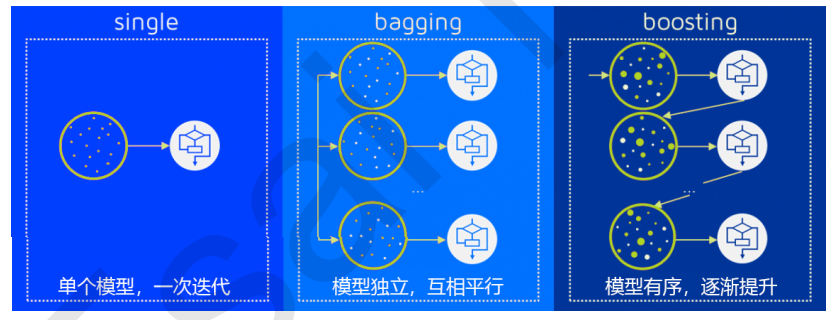# 二、重要参数

## 1、控制基评估器的参数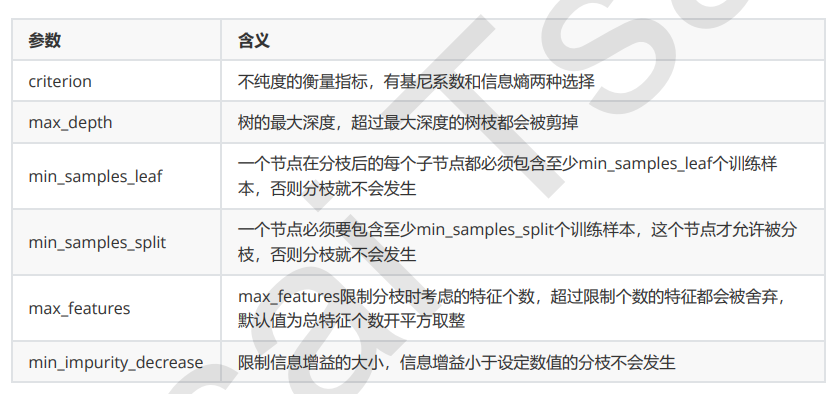单个决策树的准确率越高，随机森林的准确率也会越高，因为装袋法是依赖于平均值或 者少数服从多数原则来决定集成的结果的。

## 2、n_estimators

n_estimators越 大，模型的效果往往越好。但是相应的，任何模型都有决策边界，n_estimators达到一定的程度之后，随机森林的 精确性往往不在上升或开始波动，并且，n_estimators越大，需要的计算量和内存也越大，训练的时间也会越来越 长。对于这个参数，我们是渴望在训练难度和模型效果之间取得平衡。

n_estimators的默认值在现有版本的sklearn中是10，但是在即将更新的0.22版本中，这个默认值会被修正为 100。这个修正显示出了使用者的调参倾向：要更大的n_estimators。

from sklearn.tree import DecisionTreeClassifier
from sklearn.ensemble import RandomForestClassifier
from sklearn.model_selection import train_test_split
from sklearn.model_selection import cross_val_score
from matplotlib import pyplot as plt

x_train, x_test, y_train, y_test = train_test_split(wine.data,wine.target, test_size=0.3)

rfc = RandomForestClassifier(n_estimators=25)
rfc = rfc.fit(x_train, y_train)
score = rfc.score(x_test, y_test)
print(score)
#0.9814814814814815


画出随机森林和决策树在一组交叉验证下的效果对比

rfc = RandomForestClassifier(n_estimators=25)
rfc_s = cross_val_score(rfc,wine.data,wine.target,cv=10)
clf = DecisionTreeClassifier()
clf_s = cross_val_score(clf,wine.data,wine.target,cv=10)
plt.plot(range(1,11),rfc_s,label = "RandomForest")
plt.plot(range(1,11),clf_s,label = "Decision Tree")
plt.legend()
plt.show()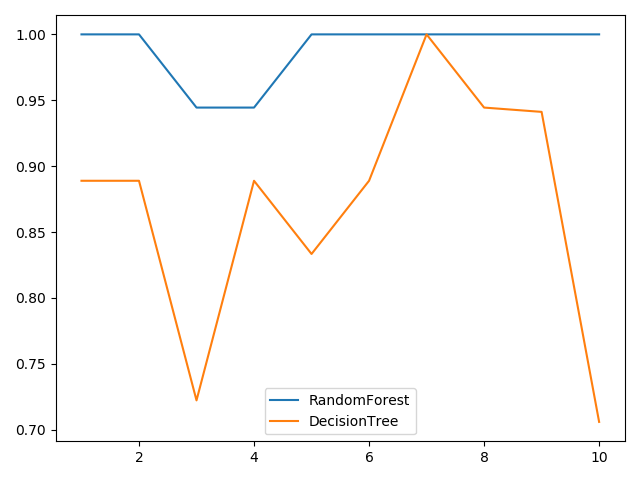画出随机森林和决策树在十组交叉验证下的效果对比

rfc_l = []
clf_l = []
for i in range(10):
rfc = RandomForestClassifier(n_estimators=25)
rfc_s = cross_val_score(rfc,wine.data,wine.target,cv=10).mean()
rfc_l.append(rfc_s)
clf = DecisionTreeClassifier()
clf_s = cross_val_score(clf,wine.data,wine.target,cv=10).mean()
clf_l.append(clf_s)

plt.plot(range(1,11),rfc_l,label = "Random Forest")
plt.plot(range(1,11),clf_l,label = "Decision Tree")
plt.legend()
plt.show()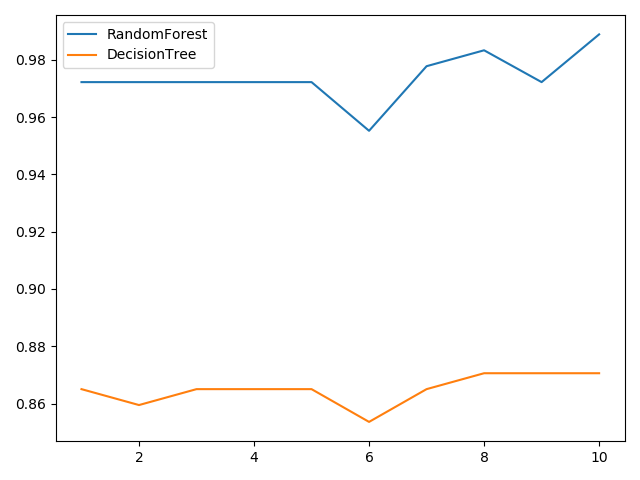n_estimators的学习曲线

superpa = []
for i in range(200):
rfc = RandomForestClassifier(n_estimators=i+1, n_jobs=-1)
rfc_s = cross_val_score(rfc, wine.data, wine.target, cv=10).mean()
superpa.append(rfc_s)
print(max(superpa), superpa.index(max(superpa)))
#0.9888888888888889 23
plt.figure(figsize=[20, 5])
plt.plot(range(1, 201), superpa)
plt.show()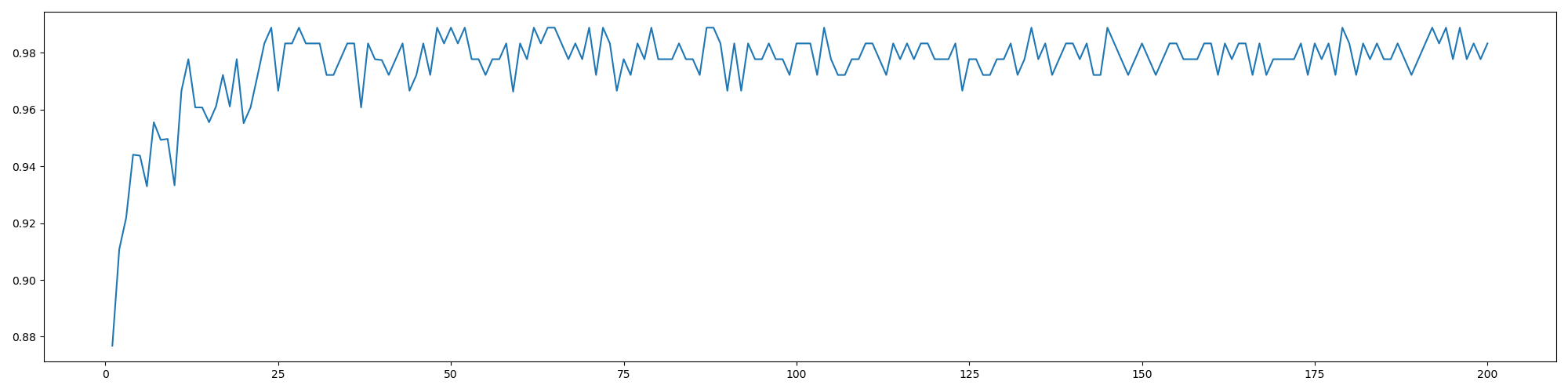## 3、random_state

随机森林的本质是一种装袋集成算法（bagging），装袋集成算法是对基评估器的预测结果进行平均或用多数表决原则来决定集成评估器的结果。在刚才的红酒例子中，我们建立了25棵树，对任何一个样本而言，平均或多数表决原则下，当且仅当有13棵以上的树判断错误的时候，随机森林才会判断错误。单独一棵决策树对红酒数据集的分类准确率在0.85上下浮动，假设一棵树判断错误的可能性为0.2(ε)，那20棵树以上都判断错误的可能性是：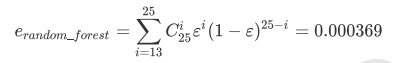可见，判断错误的几率非常小，这让随机森林在红酒数据集上的表现远远好于单棵决策树。

随机森林中其实也有random_state，用法和分类树中相似，只不过在分类树中，一个random_state只控制生成一 棵树，而随机森林中的random_state控制的是生成森林的模式，而非让一个森林中只有一棵树。

当random_state固定时，随机森林中生成是一组固定的树，但每棵树依然是不一致的，这是 用”随机挑选特征进行分枝“的方法得到的随机性。并且我们可以证明，当这种随机性越大的时候，袋装法的效果一 般会越来越好。用袋装法集成时，基分类器应当是相互独立的，是不相同的。

## 4、bootstrap & oob_score

要让基分类器尽量都不一样，一种很容易理解的方法是使用不同的训练集来进行训练，而袋装法正是通过有放回的随机抽样技术来形成不同的训练数据，bootstrap就是用来控制抽样技术的参数。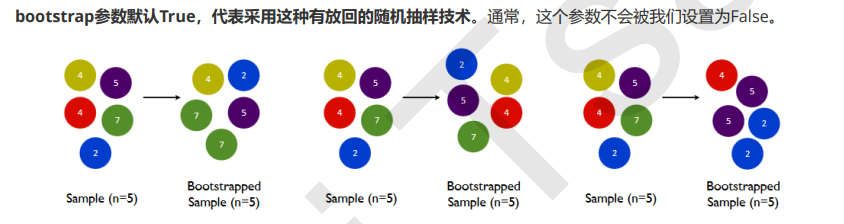一般来说，自助集大约平均会包含63%的原始数据。因为每一个样本被抽到某个自助集中的概率为：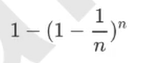当n足够大时，这个概率收敛于1-(1/e)，约等于0.632。因此，会有约37%的训练数据被浪费掉，没有参与建模， 这些数据被称为袋外数据(out of bag data，简写为oob)。

也就是说，在使用随机森林时，我们可以不划分测试集和训练集，只需要用袋外 数据来测试我们的模型即可。

#无需划分训练集和测试集然后进行交叉验证，也可以不划分，直接观察袋外数据的测试分数
rfc = RandomForestClassifier(n_estimators=25, oob_score=True)
rfc = rfc.fit(wine.data, wine.target)
#重要属性oob_score_
print(rfc.oob_score_)
#0.9662921348314607


# 三、重要属性和接口

除了.estimators_ 和 .oob_score_ 这两个重要属性。随机森林自然也有.feature_importances_这个属性。随机森林的接口与决策树完全一致，因此依然有四个常用接口：apply, fit, predict和score。除此之外，还需要注意随机森林的predict_proba接口，这个接口返回每个测试样本对应的被分到每一类标签的概率，标签有几个分类就返回几个概率。则predict_proba返回的数值大于0.5的，被分为1，小于0.5的，被分为0

rfc = RandomForestClassifier(n_estimators=25)
rfc = rfc.fit(x_train, y_train)
score = rfc.score(x_test, y_test)
print(score)
#0.9814814814814815

print(rfc.feature_importances_)  #得到所有特征值的重要性系数
print(rfc.apply(x_test))  #得到测试集所被分配到的叶子结点
rfc.predict(Xtest)
rfc.predict_proba(Xtest)


Bonus：Bagging的另一个必要条件
之前我们说过，在使用袋装法时要求基评估器要尽量独立。其实，袋装法还有另一个必要条件：基分类器的判断准确率至少要超过随机分类器，即时说，基分类器的判断准确率至少要超过50%。

import numpy as np
x = np.linspace(0,1,20)
y = []
for epsilon in np.linspace(0,1,20):
E = np.array([comb(25,i)*(epsilon**i)*((1-epsilon)**(25-i))
for i in range(13,26)]).sum()
y.append(E)
plt.plot(x,y,"o-",label="when estimators are different")
plt.plot(x,x,"--",color="red",label="if all estimators are same")
plt.xlabel("individual estimator's error")
plt.ylabel("RandomForest's error")
plt.legend()
plt.show()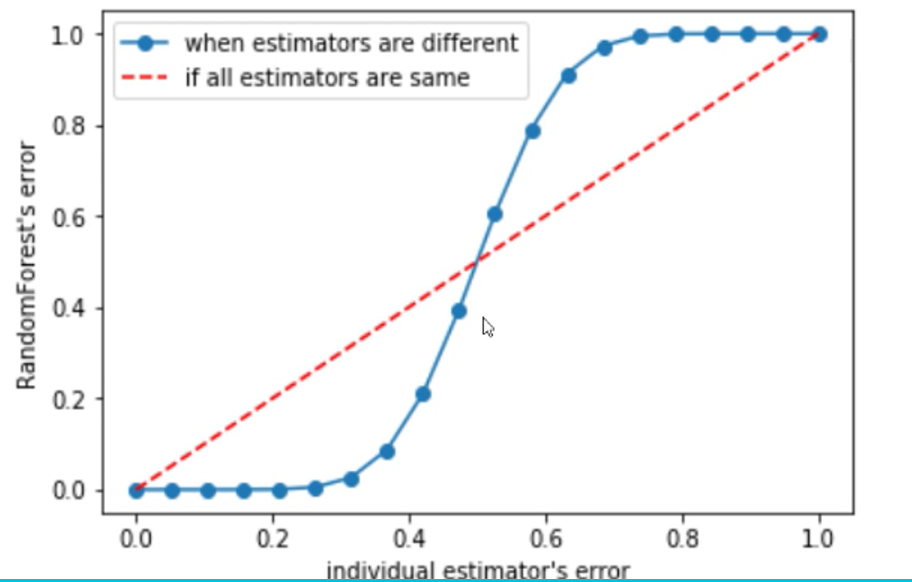# 四、随机森林回归器

## 1、重要参数，属性与接口

criterion

回归树衡量分枝质量的指标，支持的标准有三种：
1）输入"mse"使用均方误差mean squared error(MSE)，父节点和叶子节点之间的均方误差的差额将被用来作为 特征选择的标准，这种方法通过使用叶子节点的均值来最小化L2损失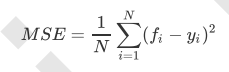2）输入“friedman_mse”使用费尔德曼均方误差，这种指标使用弗里德曼针对潜在分枝中的问题改进后的均方误差

3）输入"mae"使用绝对平均误差MAE（mean absolute error），这种指标使用叶节点的中值来最小化L1损失

在回归中，我们追求的是，MSE越小越好。 然而，回归树的接口score返回的是R平方，并不是MSE。R平方被定义如下：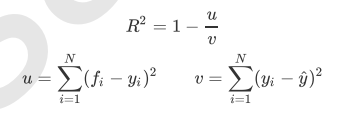虽然均方误差永远为正，但是sklearn当中使用均方误差作为评判标准时，却是计算”负均方误 差“（neg_mean_squared_error）。

最重要的属性和接口，都与随机森林的分类器相一致，还是apply, fit, predict和score最为核心。值得一提的是，随 机森林回归并没有predict_proba这个接口，因为对于回归来说，并不存在一个样本要被分到某个类别的概率问 题，因此没有predict_proba这个接口。

## 2、实例：用随机森林回归填补缺失值

我们从现实中收集的数据，几乎不可能是完美无缺的，往往都会有一些缺失值。面对缺失值，很多人选择的方式是 直接将含有缺失值的样本删除，这是一种有效的方法，但是有时候填补缺失值会比直接丢弃样本效果更好，即便我 们其实并不知道缺失值的真实样貌。我们可以使用sklearn.impute.SimpleImputer来轻松地将均 值，中值，或者其他最常用的数值填补到数据中

import numpy as np
import pandas as pd
import matplotlib.pyplot as plt
from sklearn.ensemble import RandomForestRegressor
from sklearn.impute import SimpleImputer
from sklearn.model_selection import cross_val_score

#print(boston)
x = boston.data
y = boston.target

#print(x.shape)
#(506, 13)
#print(y.shape)
#(506,)

x_full, y_full = x, y
n_samples = x_full.shape
n_features = x_full.shape

#制造缺失值
rng = np.random.RandomState(0)
missing_rate = 0.5
n_missing_samples = int(np.floor(n_samples * n_features * missing_rate))
#np.floor向下取整，返回.0格式浮点数

#所有数据要随机遍布在数据集的各行各列当中，而一个缺失的数据会需要一个行索引和一个列索引
#如果能够创造一个数组，包含3289个分布在0~506中间的行索引，和3289个分布在0~13之间的列索引，那我们就可以利用索引来为数据中的任意3289个位置赋空值
#然后我们用0，均值和随机森林来填写这些缺失值，然后查看回归的结果如何

missing_features = rng.randint(0, n_features, n_missing_samples)
missing_samples = rng.randint(0, n_samples, n_missing_samples)
#randint(起始值，终止值，产生的随机数个数）

x_missing = x_full.copy()
y_missint = y_full.copy()

x_missing[missing_samples, missing_features] = np.nan
#print(x_missing)

x_missing = pd.DataFrame(x_missing)
#print(x_missing)

#使用0和均值填补缺失值
imp_mean = SimpleImputer(missing_values=np.nan, strategy='mean')
x_missing_mean = imp_mean.fit_transform(x_missing)
#print(x_missing_mean)

#用零来填补
imp_0 = SimpleImputer(missing_values=np.nan, strategy='constant', fill_value=0)
#strategy='constant'r————>填补一个常数，数值为0
x_missing_0 = imp_mean.fit_transform(x_missing)
#print(x_missing_0)

#用随机森林回归来填补缺失值
x_missing_reg = x_missing.copy()
#print(x_missing.isnull().sum(axis=0)) 可以得到每隔列索引上的缺失值个数，但我们要的是缺失值从小到大排列的索引顺序
sort_values = np.sort(x_missing.isnull().sum(axis=0))
#print(sort_index)  #[185 189 196 197 197 200 200 201 201 202 203 204 214]得到的是缺失值个数的排列，这还不是想要的，我们要的是索引值
sort_index = np.argsort(x_missing.isnull().sum(axis=0))
#print(sort_index)  #得到了所有列缺失值个数从小到大的排列顺序，接下来要从缺失值最小的列开始，也就是按照sort_index里面的顺序开始

#建立一个新的pf_0，为了不改变原有的x_missing_reg数值

#print(pf_0) #是datafram格式

for i in sort_index:
# 构建我们的新特征矩阵和新标签
df = x_missing_reg.copy()
fillc = df.iloc[:, i]
df = pd.concat([df.iloc[:, df.columns != i], pd.DataFrame(y_full)], axis=1)

# 在新特征矩阵中，对含有缺失值的列，进行0的填补
df_0 = SimpleImputer(missing_values=np.nan,
strategy='constant', fill_value=0).fit_transform(df)

# 找出我们的训练集和测试集
Ytrain = fillc[fillc.notnull()]
Ytest = fillc[fillc.isnull()]
Xtrain = df_0[Ytrain.index, :]
Xtest = df_0[Ytest.index, :]

# 用随机森林回归来填补缺失值
rfc = RandomForestRegressor(n_estimators=100)
rfc = rfc.fit(Xtrain, Ytrain)
Ypredict = rfc.predict(Xtest)

#将填补好的特征返回到原始特征矩阵中
x_missing_reg.loc[x_missing_reg.iloc[:, i].isnull(), i] = Ypredict

#对填补好的数据进行建模
#对所有数据进行建模，取得MSE结果
X = [x_full, x_missing_mean, x_missing_0, x_missing_reg]

mse = []
for x in X:
estimator = RandomForestRegressor(n_estimators=100, random_state=0)
scorces = cross_val_score(estimator, x, y_full, cv=5, scoring='neg_mean_squared_error').mean()

mse.append(scorces * -1)

#用所得的结果画图
x_labels = ['full data', 'zero imputation', 'mean imputation', 'regressor imputation']
color = ['r', 'g', 'b', 'orange']
plt.figure(figsize=(12, 6))
ax = plt.subplot(111)
for i in range(len(x_labels)):
ax.barh(i, mse[i], color=color[i], alpha=0.6, align='center')

ax.set_title('Imputation Techniques with Boston Data')
ax.set_xlim(left=np.min(mse) * 0.9, right=np.max(mse) * 1.1)
ax.set_yticks(np.arange(len(mse)))
ax.set_xlabel('MSE')
ax.set_yticklabels(x_labels)
plt.show()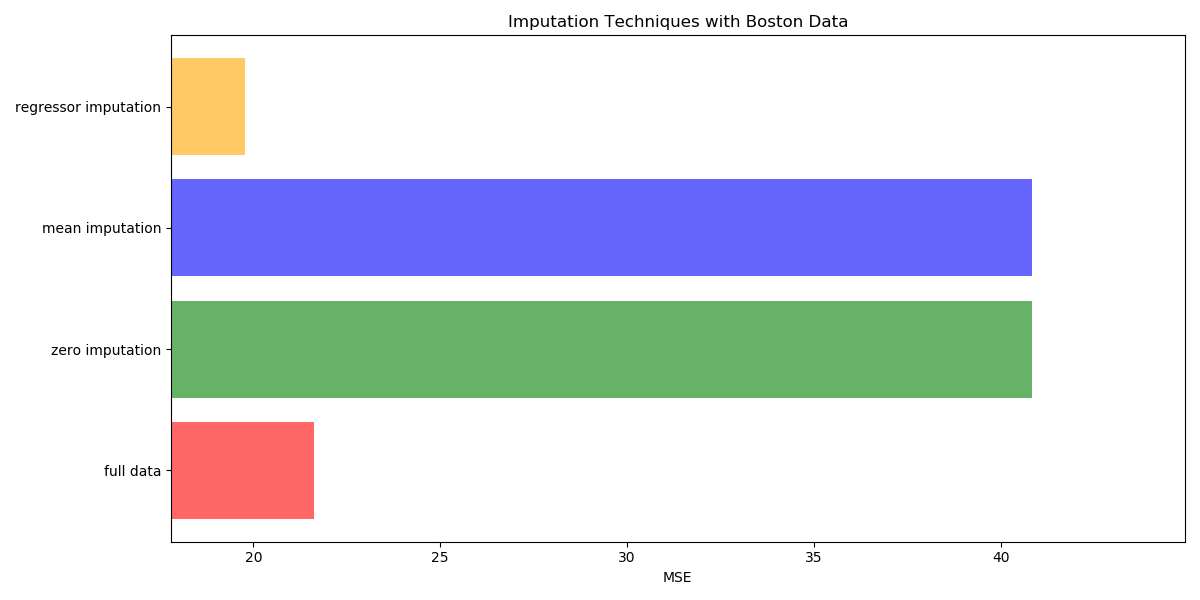# 五、机器学习中调参的基本思想

用来衡量模型在未知数据上的准确率的指标，叫做泛化误差（Genelization error）

当模型在未知数据（测试集或者袋外数据）上表现糟糕时，我们说模型的泛化程度不够，泛化误差大，模型的效果 不好。泛化误差受到模型的结构（复杂度）影响。看下面这张图，它准确地描绘了泛化误差与模型复杂度的关系， 当模型太复杂，模型就会过拟合，泛化能力就不够，所以泛化误差大。当模型太简单，模型就会欠拟合，拟合能力 就不够，所以误差也会大。只有当模型的复杂度刚刚好的才能够达到泛化误差最小的目标。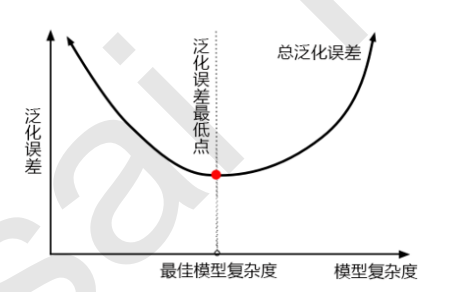1）模型太复杂或者太简单，都会让泛化误差高，我们追求的是位于中间的平衡点
2）模型太复杂就会过拟合，模型太简单就会欠拟合
3）对树模型和树的集成模型来说，树的深度越深，枝叶越多，模型越复杂
4）树模型和树的集成模型的目标，都是减少模型复杂度，把模型往图像的左边移动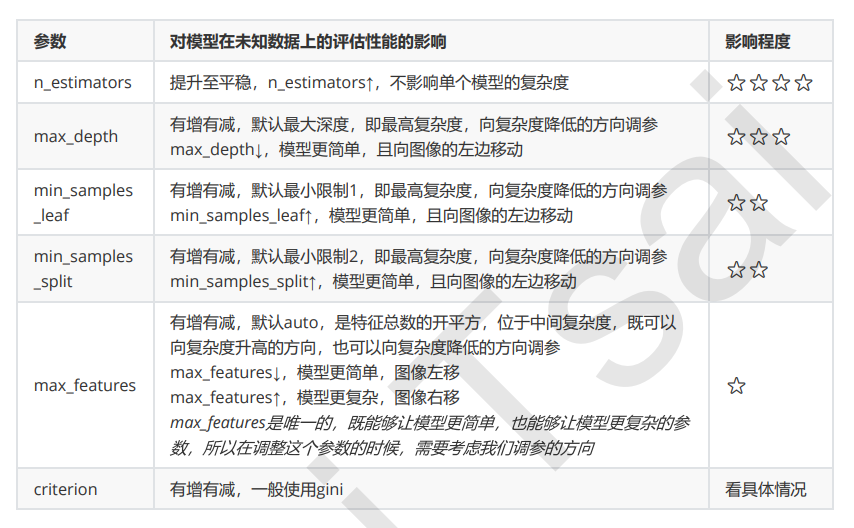# 六、 实例：随机森林在乳腺癌数据上的调参

from sklearn.datasets import load_breast_cancer
from sklearn.ensemble import RandomForestClassifier
from sklearn.model_selection import GridSearchCV
from sklearn.model_selection import cross_val_score
import pandas as pd
import numpy as np
from matplotlib import pyplot as plt

#print(x, y)
x = breast.data
y = breast.target

#单次实验结果
rfc = RandomForestClassifier()
score = cross_val_score(rfc, x, y, cv=10).mean()
print(score)  #0.9578320802005011

#画n_estimator学习曲线，选取最佳参数值
scores = []
for i in range(0, 200, 10):
rfc = RandomForestClassifier(n_estimators=i+1, n_jobs=-1, random_state=0)
#做迭代循环的时候，一定要记得加n_jobs=-1这个参数
score = cross_val_score(rfc, x, y, cv=10).mean()
scores.append(score)
#random_state=0时 0.9649122807017545 111
#random_state=90时 0.9631265664160402 71

print(max(scores), scores.index(max(scores)) * 10 + 1)
plt.figure(figsize=(16, 8))
plt.plot(range(1, 201, 10), scores)
plt.show()

#选定了random_state=0时 0.9649122807017545 111这个结果，我们可以将范围缩小到105到115之间，选定准确的数值
scores = []
for i in range(105, 116):
rfc = RandomForestClassifier(n_estimators=i, n_jobs=-1, random_state=0)
#做迭代循环的时候，一定要记得加n_jobs=-1这个参数
score = cross_val_score(rfc, x, y, cv=10).mean()
scores.append(score)
print(max(scores), [*range(105, 116)][scores.index(max(scores))])
#0.9666666666666666 110  #将n_estimators设置为110
plt.figure(figsize=(16, 8))
plt.plot(range(105, 116), scores)
plt.show()

#网格搜索，书写网格搜索的参数
#max_depth
param_grid = {'max_depth': np.arange(1, 20, 1)}
# 一般根据数据的大小来进行一个试探，乳腺癌数据很小，所以可以采用1~10，或者1~20这样的试探
# 但对于像digit recognition那样的大型数据来说，我们应该尝试30~50层深度（或许还不足够
#  更应该画出学习曲线，来观察深度对模型的影响
rfc = RandomForestClassifier(n_estimators=110, random_state=0)
GS = GridSearchCV(rfc,param_grid,cv=10)
GS.fit(x, y)
print(GS.best_params_)
print(GS.best_score_) #0.9666666666666666

#score没有变化，说明max_depth调参没有用诶，已经在最大值了

#min_samples_split
param_grid = {'min_samples_split': np.arange(2, 2+20, 1)}
rfc = RandomForestClassifier(n_estimators=110, random_state=0)
GS = GridSearchCV(rfc, param_grid, cv=10)
GS.fit(x, y)
print(GS.best_params_) #{'min_samples_split': 2}
print(GS.best_score_)  #0.9666666666666666
#说明调参没有用···

#min_samples_leaf
param_grid = {'min_samples_leaf': np.arange(1, 1+10, 1)}
rfc = RandomForestClassifier(n_estimators=110, random_state=0)
GS = GridSearchCV(rfc, param_grid, cv=10)
GS.fit(x, y)
print(GS.best_params_) #{'min_samples_leaf': 1}
print(GS.best_score_)  #0.9666666666666666 已经是最大深度了

#max_features
'''
param_grid = {'max_features': np.arange(5, 30, 1)}
rfc = RandomForestClassifier(n_estimators=110, random_state=0)
GS = GridSearchCV(rfc, param_grid, cv=10)
GS.fit(x, y)
print(GS.best_params_) #{'max_features': 7}
print(GS.best_score_)  #0.968421052631579
'''

#调整Criterion
param_grid = {'criterion': ['gini', 'entropy']}
rfc = RandomForestClassifier(n_estimators=110, random_state=0, max_features=7)
GS = GridSearchCV(rfc, param_grid, cv=10)
GS.fit(x, y)
print(GS.best_params_)  #{'criterion': 'entropy'}
print(GS.best_score_)  #0.9719298245614036

#最终参数为n_estimators=110, random_state=0, max_features=7, criterion=entropy
rfc = RandomForestClassifier(n_estimators=110, random_state=0, max_features=7, criterion='entropy')
score = cross_val_score(rfc, x, y, cv=10).mean()
print(score)
#0.9719298245614036

展开全文机器学习 sklearn python
• 行政管理支出支出总额-营销市场支出总额-创业公司运营的州利润-获利启动在应用机器学习算法之前，您必须执行以下任务：1）处理缺失值2）准备数据进行训练和测试3）应用决策树算法训练模型4）应用随机森林回归算法...
• ## 用Python实现随机森林回归

万次阅读 多人点赞 2020-11-02 13:19:38
这里就对如何进行随机森林回归在算法上进行概述，在参数上进行详述。希望对你的工作有所帮助。 这里，将介绍如何在Python中构建和使用Random Forest回归，而不是仅仅显示代码，同时将尝试了解模型的工作原理。 1.1 ...

## 1 介绍

使用Scikit-Learn模块在Python实现任何机器学习算法都比较简单，并且不需要了解所有细节。这里就对如何进行随机森林回归在算法上进行概述，在参数上进行详述。希望对你的工作有所帮助。
这里，将介绍如何在Python中构建和使用Random Forest回归，而不是仅仅显示代码，同时将尝试了解模型的工作原理。

### 1.1 随机森林概述

随机森林是一种基于集成学习的监督式机器学习算法。集成学习是一种学习类型，可以多次加入不同类型的算法或相同算法，以形成更强大的预测模型。随机森林结合了多个相同类型的算法，即多个决策的树木，故名“随机森林”。

### 1.2 理解决策树

决策树是随机森林的构建块，它本身就是个直观的模型。我们可以将决策树视为询问有关我们数据问题的流程图。这是一个可解释的模型，因为它决定了我们在现实生活中的做法：在最终得出决定之前，我们会询问有关数据的一系列问题。

随机森林是由许多决策树组成的整体模型。通过对每个决策树的预测平均来进行预测。就像森林是树木的集合一样，随机森林模型也是决策树模型的集合。这使随机森林成为一种强大的建模技术，它比单个决策树要强大得多。
随机森林中的每棵树都在对数据子集进行训练。其背后的基本思想是在确定最终输出时组合多个决策树，而不是依赖于各个决策树。每个决策树都有很高的方差，但是当我们将所有决策树并行组合在一起时，由于每个决策树都针对特定样本数据进行了完美的训练，因此结果方差很低，因此输出不依赖于一个决策树而是多个决策树木。对于回归问题，最终输出是所有决策树输出的平均值。

### 1.3 随机森林工作过程概述

1. 从数据集中随机选择N个样本子集。
2. 基于这N个样本子集构建决策树。
3. 选择算法中所需树的棵数，然后重复步骤1和2。

对于回归问题，森林中的每棵树都将预测Y值（输出）。通过取森林中所有决策树预测值的平均值来计算最终值。

### 1.4 Bootstrapping&Bagging

#### Bootstrapping

Bootstrapping算法，指的就是利用有限的样本资料经由多次重复抽样。如：在原样本中有放回的抽样，抽取n次。每抽一次形成一个新的样本，重复操作，形成很多新样本。
随机森林就是采用在每个随机样本上训练决策树。尽管每棵树相对于一组特定的训练数据可能有很大的差异，但总体而言，整个森林的方差都很小。

#### Bagging

随机森林在不同的随机选择的样本子集上训练每个决策树，然后对预测取平均以进行整体预测。这个过程被称为Bagging。

## 2 随机森林优缺点

### 2.1 随机森林回归的优点

在机器学习领域，随机森林回归算法比其他常见且流行的算法更适合回归问题。

1. 要素和标签之间存在非线性或复杂关系。
2. 它对训练集中噪声不敏感，更利于得到一个稳健的模型。随机森林算法比单个决策树更稳健，因为它使用一组不相关的决策树。
3. 避免产生过拟合的模型。

### 2.2 随机森林的缺点

1. 随机森林的主要缺点在于其复杂性。由于需要将大量决策树连接在一起，因此它们需要更多的计算资源。
2. 由于其复杂性，与其他同类算法相比，它们需要更多的时间进行训练。

### 2.3 随机森林的过拟合问题

欠拟合、合适与过拟合，如下图所示。当出现过拟合情况时候，对于训练数据模型表现良好，测试数据模型表现肯定较差。机器学习是比较容易出现过拟合的。对于随机森林的过拟合问题分为两派，会产生过拟合与不会过拟合。不会过拟合：
在Leo Breiman（随机森林算法的创建者）论文《Random Forests》中的表述：会过拟合：
问题描述，一部分数据（数据的90%）作为训练样本，一部分（10%）作为测试样本，发现训练样本的R2一直在0.85，而测试样本R2都只有0.5几，高的也就0.6几。
对于这个问题，也很有可能是自变量与因变量之间本身关系就很低产生了过拟合假象。

## 3 使用随机森林回归实践

在本节中，我们将研究如何使用Scikit-Learn将随机森林用于解决回归问题。

### 3.1 问题描述

根据汽油税（美分），人均收入（美元），高速公路（以英里为单位）和人口所占比例来预测美国48个州的汽油消耗量（百万加仑）。
为了解决这个回归问题，采用Scikit-Learn 中的随机森林算法。

### 3.2 样本数据

例子数据集可在以下位置获得（需要KeXueShangWang才可以打开）：### 3.3 代码

相关的解释说明都在代码中进行了注释

import pandas as pd
import numpy as np

# 导入数据，路径中要么用\\或/或者在路径前加r

# 输出数据预览

# 准备训练数据
# 自变量：汽油税、人均收入、高速公路、人口所占比例
# 因变量：汽油消耗量
X = dataset.iloc[:, 0:4].values
y = dataset.iloc[:, 4].values

# 将数据分为训练集和测试集
from sklearn.model_selection import train_test_split

X_train, X_test, y_train, y_test = train_test_split(X,
y,
test_size=0.2,
random_state=0)

# 特征缩放，通常没必要
# 因为数据单位，自变量数值范围差距巨大，不缩放也没问题
from sklearn.preprocessing import StandardScaler

sc = StandardScaler()
X_train = sc.fit_transform(X_train)
X_test = sc.transform(X_test)

# 训练随机森林解决回归问题
from sklearn.ensemble import RandomForestRegressor

regressor = RandomForestRegressor(n_estimators=200, random_state=0)
regressor.fit(X_train, y_train)
y_pred = regressor.predict(X_test)

# 评估回归性能
from sklearn import metrics

print('Mean Absolute Error:', metrics.mean_absolute_error(y_test, y_pred))
print('Mean Squared Error:', metrics.mean_squared_error(y_test, y_pred))
print('Root Mean Squared Error:',
np.sqrt(metrics.mean_squared_error(y_test, y_pred)))



输出结果

  Petrol_tax  Average_income  Paved_Highways  Population_Driver_licence(%)  Petrol_Consumption
0         9.0            3571            1976                         0.525                 541
1         9.0            4092            1250                         0.572                 524
2         9.0            3865            1586                         0.580                 561
3         7.5            4870            2351                         0.529                 414
4         8.0            4399             431                         0.544                 410
Mean Absolute Error: 48.33899999999999
Mean Squared Error: 3494.2330150000003
Root Mean Squared Error: 59.112037818028234


## 4 Sklearn随机森林回归参数详解

要了解sklearn.ensemble.RandomForestRegressor每个参数的意义，我们需要从函数定义入手，具体介绍还得看官网介绍：

sklearn.ensemble.RandomForestRegressor(
n_estimators=100, *, 				# 树的棵树，默认是100
criterion='mse', 					# 默认“ mse”，衡量质量的功能，可选择“mae”。
max_depth=None, 					# 树的最大深度。
min_samples_split=2, 				# 拆分内部节点所需的最少样本数：
min_samples_leaf=1, 				# 在叶节点处需要的最小样本数。
min_weight_fraction_leaf=0.0, 		# 在所有叶节点处的权重总和中的最小加权分数。
max_features='auto', 				# 寻找最佳分割时要考虑的特征数量。
max_leaf_nodes=None, 				# 以最佳优先方式生长具有max_leaf_nodes的树。
min_impurity_decrease=0.0, 			# 如果节点分裂会导致杂质的减少大于或等于该值，则该节点将被分裂。
min_impurity_split=None, 			# 提前停止树木生长的阈值。
bootstrap=True, 					# 建立树木时是否使用bootstrap抽样。 如果为False，则将整个数据集用于构建每棵决策树。
oob_score=False, 					# 是否使用out-of-bag样本估算未过滤的数据的R2。
n_jobs=None, 						# 并行运行的Job数目。
random_state=None, 					# 控制构建树时样本的随机抽样
verbose=0, 							# 在拟合和预测时控制详细程度。
warm_start=False, 					# 设置为True时，重复使用上一个解决方案，否则，只需拟合一个全新的森林。
ccp_alpha=0.0,
max_samples=None)					# 如果bootstrap为True，则从X抽取以训练每个决策树。


## 5 随机森林可视化

### 5.1 代码

import sklearn.datasets as datasets
import pandas as pd
from sklearn.model_selection import train_test_split
from sklearn.preprocessing import StandardScaler
from sklearn.pipeline import Pipeline
from sklearn.ensemble import RandomForestRegressor
from sklearn.decomposition import PCA

# 导入数据，路径中要么用\\或/或者在路径前加r

# 输出数据预览

# 准备训练数据
# 自变量：汽油税、人均收入、高速公路、人口所占比例
# 因变量：汽油消耗量
X = dataset.iloc[:, 0:4].values
y = dataset.iloc[:, 4].values

# 将数据分为训练集和测试集
X_train, X_test, y_train, y_test = train_test_split(X,
y,
test_size=0.2,
random_state=0)
regr = RandomForestRegressor()
# regr = RandomForestRegressor(random_state=100,
#                              bootstrap=True,
#                              max_depth=2,
#                              max_features=2,
#                              min_samples_leaf=3,
#                              min_samples_split=5,
#                              n_estimators=3)
pipe = Pipeline([('scaler', StandardScaler()), ('reduce_dim', PCA()),
('regressor', regr)])
pipe.fit(X_train, y_train)
ypipe = pipe.predict(X_test)

from six import StringIO
from IPython.display import Image
from sklearn.tree import export_graphviz
import pydotplus
import os

# 执行一次
# os.environ['PATH'] = os.environ['PATH']+';'+r"D:\CLibrary\Graphviz2.44.1\bin\graphviz"
dot_data = StringIO()
export_graphviz(pipe.named_steps['regressor'].estimators_,
out_file=dot_data)
graph = pydotplus.graph_from_dot_data(dot_data.getvalue())
graph.write_png('tree.png')
Image(graph.create_png())



参考博文参考博文### 5.4 随机森林可视化

X, X, X, X, X…分别为对应的自变量。 从结构化可以看到mse逐渐减小。这里一共是12层树，实际工作中可能远大于这里的例子。### 5.5 变量重要性

通过变量重要性评价，可以删除那些不重要的变量，并且性能不会受到影响。另外，如果我们使用不同的机器学习方法（例如支持向量机），则可以将随机森林特征重要性用作一种特征选择方法。

为了量化整个随机森林中所有变量对模型的贡献，我们可以查看变量的相对重要性。 Skicit-learn中返回的重要性表示包含特定变量可以提高预测。 重要性的实际计算超出了本文的范围，这里仅对模型输出重要性数值进行使用。

# Get numerical feature importances
importances = list(regr.feature_importances_)
# List of tuples with variable and importance
print(importances)

# Saving feature names for later use
feature_list = list(dataset.columns)[0:4]

feature_importances = [(feature, round(importance, 2)) for feature, importance in zip(feature_list, importances)]
# Sort the feature importances by most important first
feature_importances = sorted(feature_importances, key = lambda x: x, reverse = True)
# Print out the feature and importances
# [print('Variable: {:20} Importance: {}'.format(*pair)) for pair in feature_importances];

# Import matplotlib for plotting and use magic command for Jupyter Notebooks

import matplotlib.pyplot as plt
# Set the style
# plt.style.use('fivethirtyeight')
# list of x locations for plotting
x_values = list(range(len(importances)))
print(x_values)
# Make a bar chart
plt.bar(x_values, importances, orientation = 'vertical')
# Tick labels for x axis
plt.xticks(x_values, feature_list,rotation=6)
# Axis labels and title
plt.ylabel('Importance'); plt.xlabel('Variable'); plt.title('Variable Importances');
plt.show()展开全文• 文章目录前言一、重要参数criterion二、重要属性和接口三、随机森林回归用法总结 前言 所有的参数，属性与接口，全部和随机森林分类器一致。仅有的不同就是回归树与分类树的不同，不纯度的指标，参数Criterion不...sklearn 机器学习
• # 5 模型评估 # 随机森林回归模型评估 print("随机森林回归的默认评估值为：", rfr.score(x_test, y_test)) print("随机森林回归的R_squared值为：", r2_score(y_test, rfr_y_predict)) print("随机森林回归的均方...机器学习 python
• ## python随机森林回归数据实战

千次阅读 多人点赞 2020-11-12 11:51:53
文章目录前言一、随机森林回归器参数介绍二、数据实战1.数据介绍2.重点代码2.1特征集和标签集获取2.2数据集划分2.3随机森林模型训练2.4预测结果可视化2.5 特征重要性选择及可视化3.完整代码总结 前言 我为什么写这...机器学习 python 决策树
• 随机森林回归模型能够通过组合不同的决策树降低方差，但有时会略微增加偏差。在实际应用中，方差降低通常比偏差增加更加显著，所以随机森林回归模型能够取得更好的效果。 二.用法和参数 n_estimators参数：用于设置...算法 sklearn 机器学习
• 一、前言回归随机森林作为一种机器学习和数据分析领域常用且有效的算法，对其原理和代码实现过程的掌握是非常有必要的。为此，本文将着重介绍从零开始实现回归随机森林的过程，对于随机森林和决策树的相关理论原理将......